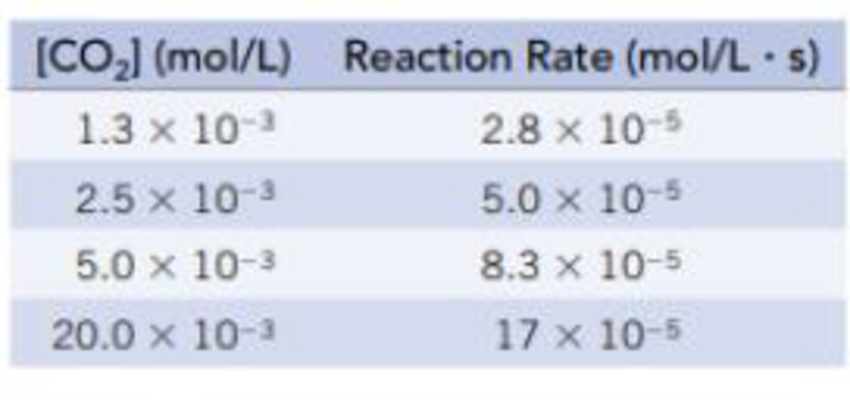Chapter 14, Problem 46PS

Chapter
Section
Textbook Problem

The enzyme carbonic anhydrase catalyzes the transformation of carbon dioxide into hydrogen carbonate ions. This reaction was studied by H. DeVoe and G. B. Kistiakowsky (Journal of the American Chemical Society, Vol. 83, p. 274, 1961) and found to obey the Michaelis-Menten model. Use the data below at a given temperature to calculate the maximum rate of the reaction. Ratemax. See Question 45 for the graphical method to use.Interpretation Introduction

Interpretation:

Using the given data the maximum rate for the given reaction has to be calculated.

Concept introduction:

In order to establish the plausibility of a mechanism, one must compare the rate law of the rate determining step to the experimentally determined rate law.

Rate determining step: In a chemical reaction the rate determining step is the slowest step in which the rate of the reaction depends on the rate of that slowest step.

Activation energy: It is defined as the minimum energy required by the reacting species in order to undergo chemical reaction.

Reactant: In a chemical reaction the species that present left is denoted as reactant which undergoes chemical change and result to given new species called product.

Product: In a chemical reaction the species that present in right side is denoted as product that results from the reactant.

Reaction coordinate: It is the diagrammatic representation of a chemical reaction which depicts how the reactants get transformed into product where the transition state and the intermediates present in the reaction are also depicted.

Enthalpy change: The change in the energy as the product formed from the reactants is represented by the enthalpy change in the reaction coordinate diagram.

Intermediate species: It is the species formed during the middle of the chemical reaction between the reactant and the desired product.

Maximum reaction rate: It is obtained by plotting inverse of concentration of the reactant with the inverse of respective rate, the point where inverse of concentration becomes 0 is the point of inverse of maximum rate which is then reversed in order to obtain the maximum rate.

Explanation

It is given that maximum rate is obtained plotting inverse of concentration of the reactant with the inverse of respective rate, the point where inverse of concentration becomes 0 is the point of inverse of maximum rate which is then reversed in order to obtain the maximum rate.

First, the given rate data in mol/L.s units should be converted into m mol/min unit by multiplying each data with 103×60 since, one mole equals to 103mmol and one minute equals to 60 seconds which then the obtained data should be inversed in order to obtain the 1[Rate]mmol/min values. Similarly, the inversion of given concentration values gives 1[S]mol/L data which are tabulated as below

Still sussing out bartleby?

Check out a sample textbook solution.

See a sample solution

The Solution to Your Study Problems

Bartleby provides explanations to thousands of textbook problems written by our experts, many with advanced degrees!

Get Started